Command - Prb_Measure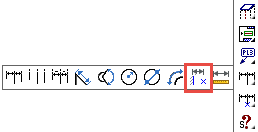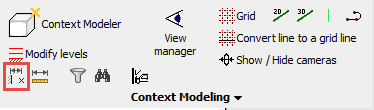With this command, you can measure the distance between 2 (sub) geometries.
The measurement will stay visible in the drawing after the command, and the value will be updated when one of the sub-geometries has changed.
The following sub-geometries are currently supported for measuring the distance between :

• Points
• Lines
• Circles
• Planar surfaces

You can use any combination of these geometries, for example line vs point or plane vs plane.

The command will ask you to select the 2 sub-geometries, after which the distance is immediately drawn on screen.
This command will actually create a new macro to do the measurement.

The measurement will always occur relative to the selected geometry.
This makes measurements on sloped geometry effortless.

By choosing the right geometry, we can do a lot of different measurements with just a few mouse clicks.
We can usually achieve the best measuring result by measuring between a planar surface and a point geometry.

Here are some examples of how choosing the right geometry can influence the measurement.
This is a profile with a sloped ending cut.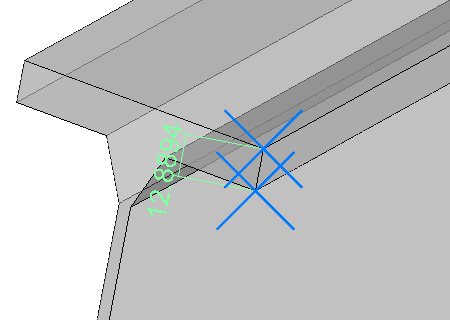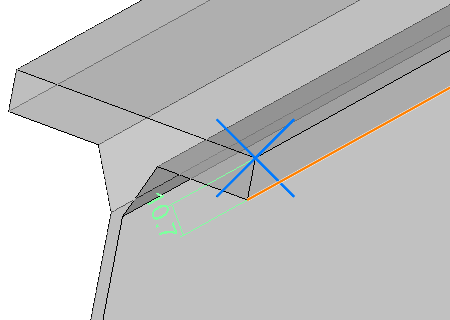Measurement between 2 points.The measurement direction is determined by the 2 points, which means that the flange thickness along the slope of the cut is measured. Measurement between a line and a point.The measurement direction is determined by the line, which means the distance value is simply the flange thickness.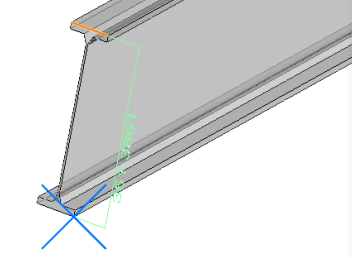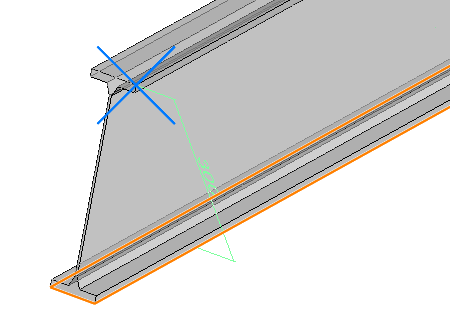Measurement between a line and a point.The measurement direction is determined by the line, which means that the total distance along the slope of the cut is measured. Measurement between a plane and a point.The measurement direction is determined by the plane, which means the distance value is simply the height of the I shape.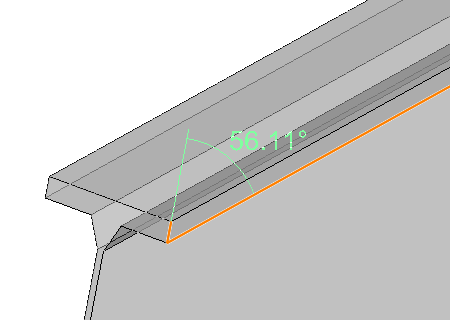Measurement between 2 lines that are not parallel.When this command is used on geometries that are not parallel, then an angle dimension is drawn instead of a distance dimension.A line to plane angle dimension is also supported.

The location of the new measurement value may not always be that obvious, especially when measuring between 2 large geometries.
The value is usually drawn in the middle of the selected geometry.

Sometimes the dimension may be drawn inside a part.
Changing the visual style to X-Ray or 2D wireframe will make the dimension visible in those cases.